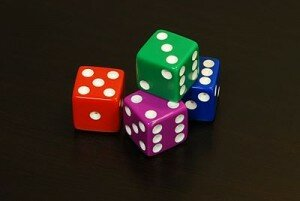# Writing Mathematical RatiosComparing numbers and quantities can be an advanced component of teaching Math, but can also be easily introduced to younger students in first or second grade. In Math, it is important to provide all students with mathematical vocabulary, like ratio or proportion. (Dice; downloaded Feb. 7, 2014; http://en.wikipedia.org/wiki/File:6sided_dice.jpg)  A ratio is nothing more than a comparison of two quantities and we use ratios even outside of the classroom. For example, when cooking, we may use 2 parts flour to 1 part sugar, that would be the ratio 2 : 1 or when mixing cleaning products, like 1/3 cup of bleach to 1 gallon of water. It is essential to explain complex terms like ratio or proportion in easy to understand language, and when possible using hands-on everyday items.

In this activity students will learn how to calculate and write a ratio.

Materials:

5 sealable bags, red/blue/green marbles or unit blocks (20 of each color), white board, dry erase markers *This is best done as a group activity with no more than 4 students per group. Prior to the activity, place a different amount of each marble color in each sealable bag. For example, Bag 1 may have 3 green, 4 blue, and 5 red. Label each bag with a number to better keep track of the bags and marbles used.

Instructions

1. Assemble the class into small groups.
2. Give each group a labeled bag of marbles.
3. Have the students count the total number of marbles in the bag and the number of marbles of each color and record the data on a piece of paper.
4. Have the groups rotate bags so that each group gets a new sealable bag and repeat steps 2 and 3.
5. Ask the students: “What did you notice about the number of marbles in each bag? What about the number of each color?”
6. Explain to the students that the number of each color marble can be written as a ratio or a comparison. You may consider saying, “A ratio is a number that compares to items together. For example, in Bag 1, there were 3 blue marbles and 5 red marbles, so we could compare or write a ratio of blue marbles to red marbles by writing 3 : 5.”

For older students, consider extending the activity by providing them with word problems involving ratios. Have them explore how ratios can be maintained by doubling or halving quantities. For example, you might ask, “if the marbles are to stay in a 3 : 1 ratio, how many marbles of each color would you need if you wanted to double the total number of marbles in Bag 3?”

Ratio activities can be a great tool to teach fractions in the classroom. For more creative math teaching tips, visit: http://nrich.maths.org/4825 and http://mathsnacks.com/teacher.php .

For more fun and interesting Learning Math Games, you can visit us here:
http://www.math-lessons.ca/activities/FractionsBoard5.html
http://www.math-lessons.ca/timestables/times-tables.html
http://www.math-lessons.ca/activities/FractionsBoard4.html
http://www.math-lessons.ca/index.html